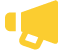Some test text!

chevron_right C++ samples

# Search PDF files for text in C++

Sample C++ code for using PDFTron SDK to search text on PDF pages using regular expressions. The TextSearch utility class builds on functionality available in TextExtractor to simplify most common search operations. Learn more about our PDF Indexed Search Library.

To run this sample, get started with a free trial of PDFTron SDK.

``````//---------------------------------------------------------------------------------------
// Consult legal.txt regarding legal and license information.
//---------------------------------------------------------------------------------------

// This sample shows how to use pdftron.PDF.TextSearch to search text on PDF pages
// using regular expressions. TextSearch utility class builds on functionality
// available in TextExtractor to simplify most common search operations.

#include <PDF/PDFNet.h>
#include <PDF/PDFDoc.h>
#include <PDF/TextSearch.h>
#include <PDF/Annot.h>
#include <iostream>

using namespace std;
using namespace pdftron;
using namespace PDF;
using namespace SDF;
using namespace Common;

#undef max
#undef min
#include <algorithm>

int main(int argc, char *argv[])
{
int ret = 0;
PDFNet::Initialize();
std::string input_path =  "../../TestFiles/credit card numbers.pdf";
const char* filein = argc>1 ? argv : input_path.c_str();

try
{
PDFDoc doc(filein);
doc.InitSecurityHandler();

TextSearch txt_search;
TextSearch::Mode mode = TextSearch::e_whole_word | TextSearch::e_page_stop;
UString pattern( "joHn sMiTh" );

//call Begin() method to initialize the text search.
txt_search.Begin( doc, pattern, mode );

int step = 0;

//call Run() method iteratively to find all matching instances.
while ( true )
{
SearchResult result = txt_search.Run();

if ( result )
{
if ( step == 0 )
{	// Step 0: found "John Smith"
// note that, here, 'ambient_string' and 'hlts' are not written to,
// as 'e_ambient_string' and 'e_highlight' are not set.

cout << result.GetMatch() << "'s credit card number is: " << endl;

//now switch to using regular expressions to find John's credit card number
mode = txt_search.GetMode();
mode |= TextSearch::e_reg_expression | TextSearch::e_highlight;
txt_search.SetMode(mode);
pattern = "\\d{4}-\\d{4}-\\d{4}-\\d{4}"; //or "(\\d{4}-){3}\\d{4}"
txt_search.SetPattern(pattern);

++step;
}
else if ( step == 1 )
{
//step 1: found John's credit card number
cout << "  " << result.GetMatch() << endl;

//note that, here, 'hlts' is written to, as 'e_highlight' has been set.
//output the highlight info of the credit card number.
Highlights hlts = result.GetHighlights();
hlts.Begin(doc);
while ( hlts.HasNext() )
{
cout << "The current highlight is from page: " << hlts.GetCurrentPageNumber() << endl;
hlts.Next();
}

//see if there is an AMEX card number
pattern = "\\d{4}-\\d{6}-\\d{5}";
txt_search.SetPattern(pattern);

++step;
}
else if ( step == 2 )
{
//found an AMEX card number
cout << "\nThere is an AMEX card number:\n  " << result.GetMatch() << endl;

//change mode to find the owner of the credit card; supposedly, the owner's
//name proceeds the number
mode = txt_search.GetMode();
mode |= TextSearch::e_search_up;
txt_search.SetMode(mode);
pattern = "[A-z]++ [A-z]++";
txt_search.SetPattern(pattern);

++step;
}
else if ( step == 3 )
{
//found the owner's name of the AMEX card
cout << "Is the owner's name:\n  " << result.GetMatch() << "?\n" << flush;

//add a link annotation based on the location of the found instance
Highlights hlts = result.GetHighlights();
hlts.Begin(doc);
while ( hlts.HasNext() )
{
Page cur_page= doc.GetPage(hlts.GetCurrentPageNumber());
for ( int i = 0; i < quad_count; ++i )
{
//assume each quad is an axis-aligned rectangle
const double *q = &quads[8*i];
double x1 = min(min(min(q, q), q), q);
double x2 = max(max(max(q, q), q), q);
double y1 = min(min(min(q, q), q), q);
double y2 = max(max(max(q, q), q), q);
Annots::Link hyper_link = Annots::Link::Create(doc, Rect(x1, y1, x2, y2), Action::CreateURI(doc, "http://www.pdftron.com"));
}
hlts.Next();
}
std::string output_path = "../../TestFiles/Output/";
doc.Save((output_path + "credit card numbers_linked.pdf").c_str(), SDFDoc::e_linearized, 0);
break;
}
}
else if ( result.IsPageEnd() )
{
//you can update your UI here, if needed
}
else
{
assert (result.IsDocEnd());
break;
}
}
}
catch(Exception& e)
{
cout << e << endl;
ret = 1;
}
catch(...)
{
cout << "Unknown Exception" << endl;
ret = 1;
}

PDFNet::Terminate();
return ret;
}``````
close

## Free Trial

Get unlimited trial usage of PDFTron SDK to bring accurate, reliable, and fast document processing capabilities to any application or workflow.

Select a platform to get started with your free trial.

Web

Android

iOS

Windows

Linux

Unlimited usage. No email address required.PDFTron Receives USD\$71 Million Growth Investment Led By Silversmith Capital Partners